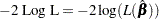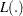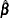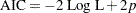### Model Fit Statistics

Suppose the model containsestimable parameters. Then the following two criteria are displayed for model fit statistics:

•2 log likelihood:whereis a partial pseudo-likelihood function for the corresponding TIES= option as described in the section Partial Likelihood Function for the Cox Model, andis the maximum pseudo-log-likelihood estimate of the proportional hazards regression coefficients.

• Akaike’s information criterion (AIC):The AIC statistics gives a different way of adjusting the2 log likelihood statistic for the number of estimable parameters in the model.